# Solution assignment 04 Logarithmic functions and graphs

### Assignment 4

Under which conditions is the following logarithmic function defined?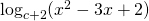### Solution

First the base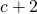has to satisfy: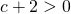and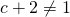so: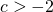and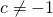Further the argument has to satisfy: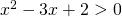The graph of the function at the left-hand side is an 'opens up' parabola and the function can be factorized: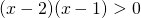The parabola lies above the-axis if: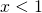or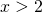and for these values ofthe logarithm is defined.

0